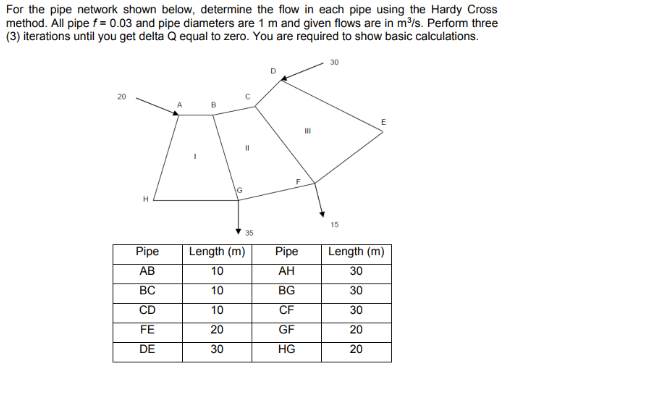## Determine the flow in each pipe using the Hardy Cross method?Transcript:
For the pipe network shown below, determine the flow in each pipe using the Hardy Cross method. All pipe f= 0.03 and pipe diameters are 1 m and given flows are in m3/s. Perform three (3) iterations until you get delta Q equal to zero. You are required to show basic calculations 30 20 15 35 Pipe Length (m Pipe Length (m) AB BC CD FE DE 10 10 10 20 30 AH BG CF GF HG 30 30 30 20 20

Solved
Fluid Mechanics 1 Answer Anonymous(s) Post